Courses

# Mathematics Notes | EduRev

## Mathematics : Mathematics Notes | EduRev

The document Mathematics Notes | EduRev is a part of the Mathematics Course Additional Topics for IIT JAM Mathematics.
All you need of Mathematics at this link: Mathematics

Functional Analysis:-
Functional analysis is a branch of mathematical analysis, the core of which is formed by the study of vector spaces endowed with some kind of limit-related structure (e.g. inner product, norm, topology, etc.) and the linear functions defined on these spaces and respecting these structures in a suitable sense. The historical roots of functional analysis lie in the study of spaces of functions and the formulation of properties of transformations of functions such as the Fourier transform as transformations defining continuous, unitary etc. operators between function spaces. This point of view turned out to be particularly useful for the study of differential and integral equations.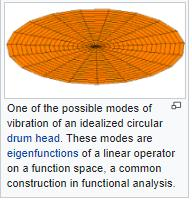The usage of the word functional as a noun goes back to the calculus of variations, implying a function whose argument is a function. The term was first used in Hadamard's 1910 book on that subject. However, the general concept of a functional had previously been introduced in 1887 by the Italian mathematician and physicist Vito Volterra. The theory of nonlinear functionals was continued by students of Hadamard, in particular Fréchet and Lévy. Hadamard also founded the modern school of linear functional analysis further developed by Riesz and the group of Polish mathematicians around Stefan Banach.
In modern introductory texts to functional analysis, the subject is seen as the study of vector spaces endowed with a topology, in particular infinite-dimensional spaces. In contrast, linear algebra deals mostly with finite-dimensional spaces, and does not use topology. An important part of functional analysis is the extension of the theory of measure, integration, and probability to infinite dimensional spaces, also known as infinite dimensional analysis.

Normed vector spaces:- The basic and historically first class of spaces studied in functional analysis are complete normed vector spaces over the real or complex numbers. Such spaces are called Banach spaces. An important example is a Hilbert space, where the norm arises from an inner product. These spaces are of fundamental importance in many areas, including the mathematical formulation of quantum mechanics.
More generally, functional analysis includes the study of Fréchet spaces and other topological vector spaces not endowed with a norm.
An important object of study in functional analysis are the continuous linear operators defined on Banach and Hilbert spaces. These lead naturally to the definition of C*-algebras and other operator algebras.

Hilbert spaces:-
Hilbert spaces can be completely classified: there is a unique Hilbert space up to isomorphism for every cardinality of the orthonormal basis. Finite-dimensional Hilbert spaces are fully understood in linear algebra, and infinite-dimensional separable Hilbert spaces are isomorphic to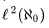. Separability being important for applications, functional analysis of Hilbert spaces consequently mostly deals with this space. One of the open problems in functional analysis is to prove that every bounded linear operator on a Hilbert space has a proper invariant subspace. Many special cases of this invariant subspace problem have already been proven.

Banach spaces:- General Banach spaces are more complicated than Hilbert spaces, and cannot be classified in such a simple manner as those. In particular, many Banach spaces lack a notion analogous to an orthonormal basis.
Examples of Banach spaces are Lp-spaces for any real number p ≥ 1. Given also a measure μ  on set X, then LP(X), sometimes also denoted LP(X,μ) or  LP(μ), has as its vectors equivalence classes [f] of measurable functions whose absolute value's p-th power has finite integral, that is, functions f, for which one has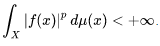If μ  is the counting measure, then the integral may be replaced by a sum. That is, we require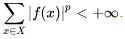Then it is not necessary to deal with equivalence classes, and the space is denoted lP(X), written more simply lp in the case when X is the set of non-negative integers.
In Banach spaces, a large part of the study involves the dual space: the space of all continuous linear maps from the space into its underlying field, so-called functionals. A Banach space can be canonically identified with a subspace of its bidual, which is the dual of its dual space. The corresponding map is an isometry but in general not onto. A general Banach space and its bidual need not even be isometrically isomorphic in any way, contrary to the finite-dimensional situation. This is explained in the dual space article.
Also, the notion of derivative can be extended to arbitrary functions between Banach spaces. See, for instance, the Fréchet derivative article.

Major and foundational results:-
Important results of functional analysis include:
Uniform boundedness principle:-
The uniform boundedness principle or Banach–Steinhaus theorem is one of the fundamental results in functional analysis. Together with the Hahn–Banach theorem and the open mapping theorem, it is considered one of the cornerstones of the field. In its basic form, it asserts that for a family of continuous linear operators (and thus bounded operators) whose domain is a Banach space, pointwise boundedness is equivalent to uniform boundedness in operator norm.
The theorem was first published in 1927 by Stefan Banach and Hugo Steinhaus but it was also proven independently by Hans Hahn.
Theorem (Uniform Boundedness Principle). Let X be a Banach space and Y be a normed vector space. Suppose that F is a collection of continuous linear operators from X to Y. If for all x in X one has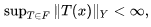then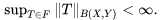Spectral theorem:-
There are many theorems known as the spectral theorem, but one in particular has many applications in functional analysis.
Theorem: Let A be a bounded self-adjoint operator on a Hilbert space H. Then there is a measure space (X, Σ, μ) and a real-valued essentially bounded measurable function f on X and a unitary operator U:H → L2μ(X) such that
where T is the multiplication operator: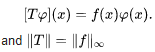This is the beginning of the vast research area of functional analysis called operator theory; see also the spectral measure.
There is also an analogous spectral theorem for bounded normal operators on Hilbert spaces. The only difference in the conclusion is that now f may be complex-valued.
Hahn–Banach theorem:-
The Hahn–Banach theorem is a central tool in functional analysis. It allows the extension of bounded linear functionals defined on a subspace of some vector space to the whole space, and it also shows that there are "enough" continuous linear functionals defined on every normed vector space to make the study of the dual space "interesting".
Hahn–Banach theorem: If p : V → R is a sublinear function, and φ : U → R is a linear functional on a linear subspace U ⊆ V which is dominated by p on U, i.e.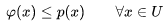then there exists a linear extension ψ : V → R of φ to the whole space V, i.e., there exists a linear functional ψ such that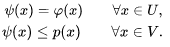Open mapping theorem:-
The open mapping theorem, also known as the Banach–Schauder theorem (named after Stefan Banach and Juliusz Schauder), is a fundamental result which states that if a continuous linear operator between Banach spaces is surjective then it is an open map. More precisely,:
Open mapping theorem. If X and Y are Banach spaces and A : X → Y is a surjective continuous linear operator, then A is an open map (i.e. if U is an open set in X, then A(U) is open in Y).
The proof uses the Baire category theorem, and completeness of both X and Y is essential to the theorem. The statement of the theorem is no longer true if either space is just assumed to be a normed space, but is true if X and Y are taken to be Fréchet spaces.
Closed graph theorem:-
The closed graph theorem states the following: If X is a topological space and Y is a compact Hausdorff space, then the graph of a linear map T from X to Y is closed if and only if T is continuous.

Foundations of mathematics considerations:-
Most spaces considered in functional analysis have infinite dimension. To show the existence of a vector space basis for such spaces may require Zorn's lemma. However, a somewhat different concept, Schauder basis, is usually more relevant in functional analysis. Many very important theorems require the Hahn–Banach theorem, usually proved using axiom of choice, although the strictly weaker Boolean prime ideal theorem suffices. The Baire category theorem, needed to prove many important theorems, also requires a form of axiom of choice.

Points of view:-

Functional analysis in its present form includes the following tendencies:

• Abstract analysis. An approach to analysis based on topological groups, topological rings, and topological vector spaces.
• Geometry of Banach spaces contains many topics. One is combinatorial approach connected with Jean Bourgain; another is a characterization of Banach spaces in which various forms of the law of large numbers hold.
• Noncommutative geometry. Developed by Alain Connes, partly building on earlier notions, such as George Mackey's approach to ergodic theory.
• Connection with quantum mechanics. Either narrowly defined as in mathematical physics, or broadly interpreted by, e.g. Israel Gelfand, to include most types of representation theory.
Offer running on EduRev: Apply code STAYHOME200 to get INR 200 off on our premium plan EduRev Infinity!

40 docs

,

,

,

,

,

,

,

,

,

,

,

,

,

,

,

,

,

,

,

,

,

;